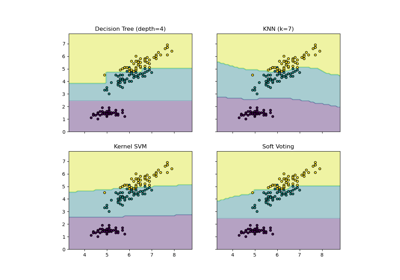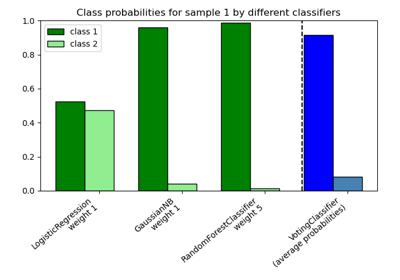# sklearn.ensemble.VotingClassifier¶

class sklearn.ensemble.VotingClassifier(estimators, *, voting='hard', weights=None, n_jobs=None, flatten_transform=True, verbose=False)[source]

Soft Voting/Majority Rule classifier for unfitted estimators.

New in version 0.17.

Read more in the User Guide.

Parameters
estimatorslist of (str, estimator) tuples

Invoking the fit method on the VotingClassifier will fit clones of those original estimators that will be stored in the class attribute self.estimators_. An estimator can be set to 'drop' using set_params.

Changed in version 0.21: 'drop' is accepted.

Deprecated since version 0.22: Using None to drop an estimator is deprecated in 0.22 and support will be dropped in 0.24. Use the string 'drop' instead.

voting{‘hard’, ‘soft’}, default=’hard’

If ‘hard’, uses predicted class labels for majority rule voting. Else if ‘soft’, predicts the class label based on the argmax of the sums of the predicted probabilities, which is recommended for an ensemble of well-calibrated classifiers.

weightsarray-like of shape (n_classifiers,), default=None

Sequence of weights (float or int) to weight the occurrences of predicted class labels (hard voting) or class probabilities before averaging (soft voting). Uses uniform weights if None.

n_jobsint, default=None

The number of jobs to run in parallel for fit. None means 1 unless in a joblib.parallel_backend context. -1 means using all processors. See Glossary for more details.

New in version 0.18.

flatten_transformbool, default=True

Affects shape of transform output only when voting=’soft’ If voting=’soft’ and flatten_transform=True, transform method returns matrix with shape (n_samples, n_classifiers * n_classes). If flatten_transform=False, it returns (n_classifiers, n_samples, n_classes).

verbosebool, default=False

If True, the time elapsed while fitting will be printed as it is completed.

Attributes
estimators_list of classifiers

The collection of fitted sub-estimators as defined in estimators that are not ‘drop’.

named_estimators_Bunch

Attribute to access any fitted sub-estimators by name.

New in version 0.20.

classes_array-like of shape (n_predictions,)

The classes labels.

VotingRegressor

Prediction voting regressor.

Examples

>>> import numpy as np
>>> from sklearn.linear_model import LogisticRegression
>>> from sklearn.naive_bayes import GaussianNB
>>> from sklearn.ensemble import RandomForestClassifier, VotingClassifier
>>> clf1 = LogisticRegression(multi_class='multinomial', random_state=1)
>>> clf2 = RandomForestClassifier(n_estimators=50, random_state=1)
>>> clf3 = GaussianNB()
>>> X = np.array([[-1, -1], [-2, -1], [-3, -2], [1, 1], [2, 1], [3, 2]])
>>> y = np.array([1, 1, 1, 2, 2, 2])
>>> eclf1 = VotingClassifier(estimators=[
...         ('lr', clf1), ('rf', clf2), ('gnb', clf3)], voting='hard')
>>> eclf1 = eclf1.fit(X, y)
>>> print(eclf1.predict(X))
[1 1 1 2 2 2]
>>> np.array_equal(eclf1.named_estimators_.lr.predict(X),
...                eclf1.named_estimators_['lr'].predict(X))
True
>>> eclf2 = VotingClassifier(estimators=[
...         ('lr', clf1), ('rf', clf2), ('gnb', clf3)],
...         voting='soft')
>>> eclf2 = eclf2.fit(X, y)
>>> print(eclf2.predict(X))
[1 1 1 2 2 2]
>>> eclf3 = VotingClassifier(estimators=[
...        ('lr', clf1), ('rf', clf2), ('gnb', clf3)],
...        voting='soft', weights=[2,1,1],
...        flatten_transform=True)
>>> eclf3 = eclf3.fit(X, y)
>>> print(eclf3.predict(X))
[1 1 1 2 2 2]
>>> print(eclf3.transform(X).shape)
(6, 6)


Methods

 fit(X, y[, sample_weight]) Fit the estimators. fit_transform(X[, y]) Fit to data, then transform it. get_params([deep]) Get the parameters of an estimator from the ensemble. Predict class labels for X. score(X, y[, sample_weight]) Return the mean accuracy on the given test data and labels. set_params(**params) Set the parameters of an estimator from the ensemble. Return class labels or probabilities for X for each estimator.
__init__(estimators, *, voting='hard', weights=None, n_jobs=None, flatten_transform=True, verbose=False)[source]

Initialize self. See help(type(self)) for accurate signature.

fit(X, y, sample_weight=None)[source]

Fit the estimators.

Parameters
X{array-like, sparse matrix} of shape (n_samples, n_features)

Training vectors, where n_samples is the number of samples and n_features is the number of features.

yarray-like of shape (n_samples,)

Target values.

sample_weightarray-like of shape (n_samples,), default=None

Sample weights. If None, then samples are equally weighted. Note that this is supported only if all underlying estimators support sample weights.

New in version 0.18.

Returns
selfobject
fit_transform(X, y=None, **fit_params)[source]

Fit to data, then transform it.

Fits transformer to X and y with optional parameters fit_params and returns a transformed version of X.

Parameters
X{array-like, sparse matrix, dataframe} of shape (n_samples, n_features)
yndarray of shape (n_samples,), default=None

Target values.

**fit_paramsdict

Returns
X_newndarray array of shape (n_samples, n_features_new)

Transformed array.

get_params(deep=True)[source]

Get the parameters of an estimator from the ensemble.

Parameters
deepbool, default=True

Setting it to True gets the various classifiers and the parameters of the classifiers as well.

predict(X)[source]

Predict class labels for X.

Parameters
X{array-like, sparse matrix} of shape (n_samples, n_features)

The input samples.

Returns
majarray-like of shape (n_samples,)

Predicted class labels.

property predict_proba

Compute probabilities of possible outcomes for samples in X.

Parameters
X{array-like, sparse matrix} of shape (n_samples, n_features)

The input samples.

Returns
avgarray-like of shape (n_samples, n_classes)

Weighted average probability for each class per sample.

score(X, y, sample_weight=None)[source]

Return the mean accuracy on the given test data and labels.

In multi-label classification, this is the subset accuracy which is a harsh metric since you require for each sample that each label set be correctly predicted.

Parameters
Xarray-like of shape (n_samples, n_features)

Test samples.

yarray-like of shape (n_samples,) or (n_samples, n_outputs)

True labels for X.

sample_weightarray-like of shape (n_samples,), default=None

Sample weights.

Returns
scorefloat

Mean accuracy of self.predict(X) wrt. y.

set_params(**params)[source]

Set the parameters of an estimator from the ensemble.

Valid parameter keys can be listed with get_params().

Parameters
**paramskeyword arguments

Specific parameters using e.g. set_params(parameter_name=new_value). In addition, to setting the parameters of the stacking estimator, the individual estimator of the stacking estimators can also be set, or can be removed by setting them to ‘drop’.

transform(X)[source]

Return class labels or probabilities for X for each estimator.

Parameters
X{array-like, sparse matrix} of shape (n_samples, n_features)

Training vectors, where n_samples is the number of samples and n_features is the number of features.

Returns
probabilities_or_labels
If voting='soft' and flatten_transform=True:

returns ndarray of shape (n_classifiers, n_samples * n_classes), being class probabilities calculated by each classifier.

If voting='soft' and flatten_transform=False:

ndarray of shape (n_classifiers, n_samples, n_classes)

If voting='hard':

ndarray of shape (n_samples, n_classifiers), being class labels predicted by each classifier.

## Examples using sklearn.ensemble.VotingClassifier`¶